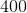## Example Questions

### Example Question #1 : How To Find The Part From The Whole

Quantitative Comparison

Quantity A: 10% of $45 Quantity B: 45% of$10

The relationship cannot be determined from the information given.

The two quantities are equal.

Quantity A is greater.

Quantity B is greater.

The two quantities are equal.

Explanation:

Quantity A: .1 * 45 = $4.50 Quantity B: .45 * 10 =$4.50

Therefore the two quantities are equal.  This is always true: a% of $b = b% of$a.

### Example Question #11 : Percentage

What percent of 5 is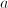?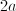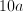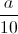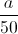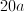Explanation: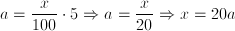### Example Question #1 : How To Find The Part From The Whole

Quantity A:, whereis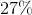ofQuantity B:, whereis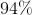ofWhich of the following is true?

The two quantities are equal.

A comparison cannot be detemined from the given information.

Quantity B is larger.

Quantity A is larger.

Quantity B is larger.

Explanation:

This type of problem is very easy.  You merely need to translate the text into the form of an equation.  For this, remember that "of" is translated as multiplication and "is" as equality.  This gives us the following.

Quantity A:isofBecomes...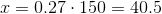Quantity B:isofBecomes...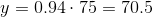Therefore, quantity B is larger.

### Example Question #1 : Whole And Part

Quantity A:, whereis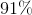of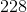Quantity B:, whereis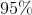of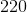Which of the following is true?

Quantity A is larger.

A comparison cannot be detemined from the given information.

The two quantities are equal.

Quantity B is larger.

Quantity B is larger.

Explanation:

This type of problem is very easy.  You merely need to translate the text into the form of an equation.  For this, remember that "of" is translated as multiplication and "is" as equality.  This gives us the following.

Quantity A:isofBecomes...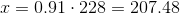Quantity B:isofBecomes...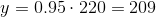Therefore, quantity B is larger.

### Example Question #2 : Whole And Part

Quantity A:, whereis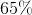of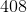Quantity B:, whereisof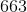Which of the following is true?

Quantity A is greater.

A comparison cannot be detemined from the given information.

The two quantities are equal.

Quantity B is greater.

The two quantities are equal.

Explanation:

This type of problem is very easy.  You merely need to translate the text into the form of an equation.  For this, remember that "of" is translated as multiplication and "is" as equality.  This gives us the following.

Quantity A:isofBecomes...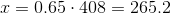Quantity B:isofBecomes...Therefore, the two quantities are equal.

### Example Question #6 : How To Find The Part From The Whole

A bag contains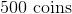.are quarters,are dimes and the rest are nickels. How much money is in the bag in nickels?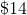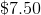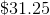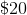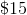Explanation:

To solve this problem we must first find what percent of the money in the bag is in nickels. We know that combined, quarters and  dimes make up 40% of the coins and that the rest are nickels. Therefore 60% of the money in the bag are nickels. We then multiply the total amount of coins in the bag with that percentage in order to find out how many nickels are in the bag.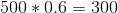.  There are 300 nickels in the bag and nickels are worth 5 cents each. Therefore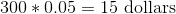worth of nickels in the bag.

### Example Question #1 : How To Find The Whole From The Part

36 is what percent of 145

403%

24.8%

40.3%

36%

2.48%

24.8%

Explanation:

divide: 36/145 = 0.248; multiply by 100 to get percent

24.8%

### Example Question #2 : How To Find The Whole From The Part

36 is x% of 133.  What is x

10

19

22.4

27

42

27

Explanation:

36 is x% of 133

that means that 36 = (x%)(133)

x% = 36/133 X 100 = 27%

### Example Question #3 : How To Find The Whole From The Part

Max walks 1 mile in 15 minutes. Belinda takes only 12 minutes to walk 1 mile. If Max and Belinda leave their homes at the same time, how far has Belinda walked when Max has walked 1 mile?

4/3 mile

3/2 mile

5/4 mile

3/4 mile

1 mile

5/4 mile

Explanation:

Belinda walks faster than Max, so she should walk over a mile in the same time that Max walks 1 mile. We can eliminate the answer choices that aren't over a mile. It takes Max 3 minutes longer to walk a mile, so Belinda will walk for 3 more minutes after she finishes her first mile. If she walks 1 mile in 12 minutes, she walks 1/12 miles in 1 minute, or 3/12 = 1/4 mile in 3 minutes. So she walks 1 1/4 miles, or 5/4 miles.

### Example Question #4 : How To Find The Whole From The Part

If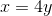is what percent of?